# Maharashtra Board 12th Commerce Maths Solutions Chapter 1 Mathematical Logic Ex 1.10

Balbharati Maharashtra State Board 12th Commerce Maths Solution Book Pdf Chapter 1 Mathematical Logic Ex 1.10 Questions and Answers.

## Maharashtra State Board 12th Commerce Maths Solutions Chapter 1 Mathematical Logic Ex 1.10

Question 1.
Express the truth of each of the following statements by Venn diagrams:
(i) Some hardworking students are obedient.
Solution:
Let U : set of all students
S : set of all hardworking students
O : set of all obedient students.
Then the Venn diagram represents the truth of the given statement is as below: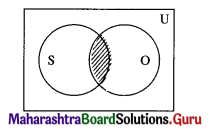S ∩ O ≠ φ

(ii) No circles are polygons.
Solution:
Let U : set of closed geometrical figures in the plane
P : set of all polygons
C : set of all circles.
Then the Venn diagram represents the truth of the given statement is as follows: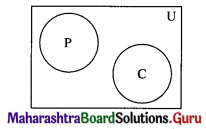P ∩ C = φ(iii) All teachers are scholars and scholars are teachers.
Solution:
Let U : set of all human beings
T : set of all teachers
S : set of all scholars.
Then the Venn diagram represents the truth of the given statement is as below: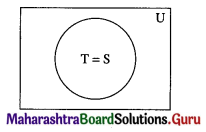(iv) If a quadrilateral is a rhombus, then it is a parallelogram.
Solution:
Let U : set of all quadrilaterals
R : set of all rhombus
P : set of all parallelograms.
Then the Venn diagram represents the truth of the given statement is as below: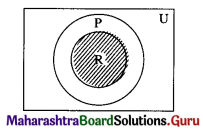R ⊂ P

Question 2.
Draw the Venn diagrams for the truth of the following statements:
(i) Some share brokers are chartered accountants.
Solution:
Let U : set of all human beings
S : set of all share brokers
C : set of all chartered accountants.
Then the Venn diagram represents the truth of the given statement is as below: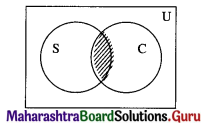S ∩ C ≠ φ

(ii) No wicket-keeper is a bowler in a cricket team.
Solution:
Let U : set of all human beings
W : set of all wicket keepers
B : set of all bowlers.
Then the Venn diagram represents the truth of the given statement is as follows: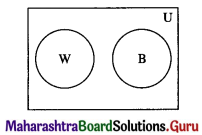W ∩ B = φQuestion 3.
Represent the following statements by Venn diagrams:
(i) Some non-resident Indians are not rich.
Solution:
Let U : set of all human beings
N : set of all non-resident Indians
R : set of all rich people.
Then the Venn diagram represents the truth of the given statement is as below: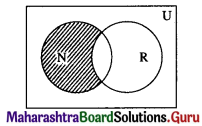N – R ≠ φ

(ii) No circle is a rectangle.
Solution:
Let U : set of all geometrical figures
C : set of all circles
R : set of all rectangles
Then the Venn diagram represents the truth of the given statement is as below: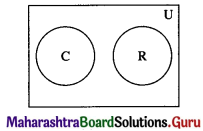C ∩ R = φ(iii) If n is a prime number and n ≠ 2, then it is odd.
Solution:
Let U : set of all real numbers
P : set of all prime numbers n, where n ≠ 2
O : set of all odd numbers.
Then the Venn diagram represents the truth of the given statement is as below: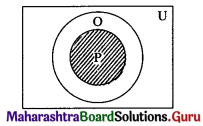P ⊂ O